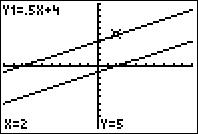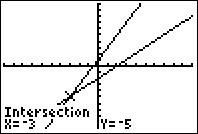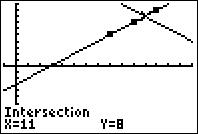# Activities

••• ##### Subject Area

• Math: Algebra I: Linear Functions

• ##### Author9-12

30 Minutes

• ##### Device
• TI-83 Plus Family
• TI-84 Plus
• TI-84 Plus Silver Edition
•TI-84 Plus C Silver Edition
•TI-84 Plus CE
• ##### Software

TI Connect™
TI Connect™ CE

• ##### Accessories

TI Connectivity Cable

• ##### Report an Issue

All On The Line#### Activity Overview

Students enter points into a spreadsheet and graph equations to help solve linear systems.

#### Key Steps

•In Problem 1, students will graph two intersecting lines and be asked to find the ordered pair for the intersection point. They will determine what the intersection point represents for the set of equations and how they can verify the point. An additional problem is provided for practice.

•In Problem 2, students are introduced to parallel lines in a system. Students will notice that when the slopes are equal, the lines are parallel and will not intersect. Problem 3 demonstrates the case where two lines with the same slope and y-intercept have infinitely many solutions.

•For the homework problems, students will write two equations (a system) for solving a word problem. In lists, they will enter three pairs of numbers which can be displayed on the graph with a Stat Plot. Once the two linear equations are entered, the graph will reveal the solution to the problem. Students can verify the solution with the Intersect tool.